# Problem 53735. Easy Sequences 58: Curious Prime-Rational Functions

For some prime numbers p and q where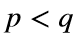, a rational function R, is defined as follows:. Using the output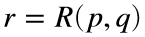, another rational function K, is defined:. Finaly, using the output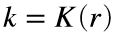, we define the function N:; where the symbol "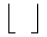", represents the integer part of the decimal expansion of the fraction k .
For example for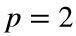and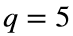: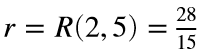;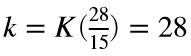; since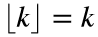,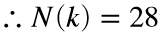.
And, for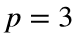and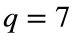: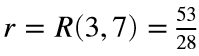;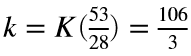; since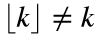,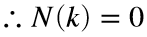.
If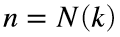, and given an integer limit x, write a function that returns a sorted array of all unique values of n that are less than or equal to x.
-----------
HINT: Both R and K, are rational functions and expect exact rational fraction outputs. Therefore, please preserve numerators and denominators for R and K, and evaluate decimal expansions only when calculating the output of the function N.

### Solution Stats

54.55% Correct | 45.45% Incorrect
Last Solution submitted on Nov 19, 2023

### Community Treasure Hunt

Find the treasures in MATLAB Central and discover how the community can help you!

Start Hunting!